Physiolibrary.Thermal.Components

Information

Extends from Modelica.Icons.Package (Icon for standard packages).

Package Content

Name Description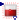HeatAccumulation Accumulating of heat to substance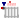IdealRadiator Closed circiut radiator, where outflowed = ambient temperature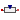Conductor Heat resistor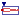Stream Flow of whole heated mass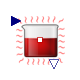Physiolibrary.Thermal.Components.HeatAccumulation

Accumulating of heat to substanceInformation

The "HeatAccumulation" library class models heat accumulation in Physiolibrary. This class has one thermal connector with temperature and heat flow. Heat energy is accumulated inside the class, stored in the variable "relativeHeat". This value is relative to normal body temperature of 37 °C; a positive value therefore indicates an internal temperature above 37 °C, while a negative value indicates temperature below 37 °C. Of course the particular value of temperature depends on the mass and specific heat of the instance.

Extends from Icons.HeatAccumulation, SteadyStates.Interfaces.SteadyState (Abstract class for any dynamic state calculation (for any derivation), which is driven by SimulationType option.).

Parameters

TypeNameDefaultDescription
SpecificHeatCapacitySpecificHeat4186.8Mass specific heat [J/(kg.K)]
MassWeight1Total mass weight if useMassInput=false [kg]
Initialization
HeatrelativeHeat_start0Heat start value = weight*(initialTemperature - 37degC)*specificHeat [J]
External inputs/outputs
BooleanuseMassInputfalse=true, if mass input is used
IO
replaceable package UtilitiesTypes.FilesUtilities (direct...How to store or load the values
Initialization
Realstate_startrelativeHeat_startState start or init value
Value I/O
StringstoreUnit"kcal"Unit in Utilities input/output function
StringstateNamegetInstanceName()Name in Utilities input/output function
BooleanSAVE_RESULTSfalseSave final state values
BooleanSAVE_COMPARISONfalseCompare final state values with original values
Equilibrium
Simulation
SimulationTypeSimulationTypes.SimulationType.NormalI...Dynamic with Initialization or Steady State
BooleanisDependentfalse=true, If zero flow is propagated in eqiulibrium through resistors, impedances, reactions, etc.

Connectors

TypeNameDescription
HeatPort_bq_inHeat inflow/outflow connector
output HeatOutputrelativeHeatCurrent accumulated heat = weight*(T - 37degC)*specificHeat [J]
input MassInputweightWeight of mass, where the heat are accumulated [kg]

Modelica definition

model HeatAccumulation "Accumulating of heat to substance" extends Icons.HeatAccumulation; extends SteadyStates.Interfaces.SteadyState( state_start=relativeHeat_start, storeUnit= "kcal"); Interfaces.HeatPort_b q_in "Heat inflow/outflow connector"; parameter Types.Heat relativeHeat_start = 0 "Heat start value = weight*(initialTemperature - 37degC)*specificHeat"; parameter Types.SpecificHeatCapacity SpecificHeat= 4186.8 "Mass specific heat"; Types.Temperature T "Current temperature"; Types.RealIO.HeatOutput relativeHeat "Current accumulated heat = weight*(T - 37degC)*specificHeat"; //nominal=1 //absoluteHeat = weight*310.15*specificHeat + relativeHeat constant Types.Temperature NormalBodyTemperature = 310.15 "Shift of absolute zero temperature to normal body values"; parameter Boolean useMassInput = false "=true, if mass input is used"; parameter Types.Mass Weight=1 "Total mass weight if useMassInput=false"; Types.RealIO.MassInput weight(start=Weight)=m if useMassInput "Weight of mass, where the heat are accumulated"; protected Types.Mass m; equation if not useMassInput then m=Weight; end if; q_in.T=NormalBodyTemperature + relativeHeat/(m*SpecificHeat); T = q_in.T; state = relativeHeat; // der(relativeHeat)=q_in.q change = q_in.Q_flow; end HeatAccumulation;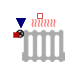Physiolibrary.Thermal.Components.IdealRadiator

Closed circiut radiator, where outflowed = ambient temperatureInformation

This class has two thermal connectors – one for liquid inside the radiator and another for the material around the radiator. Note that there is no liquid flow inside these connectors. The liquid flow can be described by a parameter or input to the instance. Together with the liquid specific heat, this flow determines the amount of heat flux from the liquid to a surrounding environment of different temperature. The calculation fulfills the ideal condition of microcirculation, where the outflowing blood has the same temperature as a tissue. This is really useful for modeling body thermal transfers, because the transfer of heat with blood flow is more significant than the typical rate of conduction through solid mass.

The real inflow to radiator can be described by substanceFlow and temperature q_in.T.

q_in.q=q_out.q is not the heat inflow to Radiator input, but the heat convected from radiator to environment!

The environment temperature is the same as radiator output temperature q_out.T.

And the flow of heat from radiator to environment is driven by Fick principle.

Extends from Interfaces.ConditionalMassFlow (Input of mass flow vs. parametric mass flow), Icons.Radiator.

Parameters

TypeNameDefaultDescription
MassFlowRateMassFlow0Mass flow if useMassFlowInput=false [kg/s]
SpecificHeatCapacitySpecificHeat3851.856Specific heat of flow circuit medium [J/(kg.K)]
External inputs/outputs
BooleanuseMassFlowInputfalse=true, if mass flow input is used instead of parameter MassFlow

Connectors

TypeNameDescription
input MassFlowRateInputmassFlow[kg/s]
HeatPort_aq_in
HeatPort_bq_out

Modelica definition

model IdealRadiator "Closed circiut radiator, where outflowed = ambient temperature" extends Interfaces.ConditionalMassFlow; extends Icons.Radiator; parameter Types.SpecificHeatCapacity SpecificHeat=3851.856 "Specific heat of flow circuit medium"; //default heat capacity of blood is used as 0.92 kcal/(degC.kg) Thermal.Interfaces.HeatPort_a q_in; Thermal.Interfaces.HeatPort_b q_out; equation q_in.Q_flow + q_out.Q_flow = 0; // assert(substanceFlow>=-Modelica.Constants.eps,"In IdealRadiator must be always the forward flow direction! Not 'substanceFlow<0'!"); q_in.Q_flow = q*(q_in.T-q_out.T)*SpecificHeat; end IdealRadiator;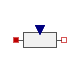Physiolibrary.Thermal.Components.Conductor

Heat resistorInformation

Extends from Interfaces.OnePort (Heat OnePort), Icons.Resistor.

Parameters

TypeNameDefaultDescription
ThermalConductanceConductance0Thermal conductance if useConductanceInput=false [W/K]
External inputs/outputs
BooleanuseConductanceInputfalse=true, if external conductance value is used

Connectors

TypeNameDescription
HeatPort_aq_in
HeatPort_bq_out
input ThermalConductanceInputconductance[W/K]

Modelica definition

model Conductor "Heat resistor" extends Interfaces.OnePort; extends Icons.Resistor; parameter Boolean useConductanceInput = false "=true, if external conductance value is used"; parameter Types.ThermalConductance Conductance=0 "Thermal conductance if useConductanceInput=false"; Types.RealIO.ThermalConductanceInput conductance(start=Conductance)=c if useConductanceInput; protected Types.ThermalConductance c; equation if not useConductanceInput then c=Conductance; end if; q_in.Q_flow = c * (q_in.T - q_out.T); end Conductor;Physiolibrary.Thermal.Components.Stream

Flow of whole heated massInformation

Bidirectional heated mass flow by temperature

Possible field values:

>=0

<=0

q_in.q

=massFlow*q_in.T*SpecificHeat

=-q_out.q

q_out.q

=-q_in.q

=massFlow*q_out.T*SpecificHeat

Extends from Interfaces.OnePort (Heat OnePort), Interfaces.ConditionalMassFlow (Input of mass flow vs. parametric mass flow).

Parameters

TypeNameDefaultDescription
MassFlowRateMassFlow0Mass flow if useMassFlowInput=false [kg/s]
SpecificHeatCapacitySpecificHeat Of flow circuit medium [J/(kg.K)]
External inputs/outputs
BooleanuseMassFlowInputfalse=true, if mass flow input is used instead of parameter MassFlow

Connectors

TypeNameDescription
HeatPort_aq_in
HeatPort_bq_out
input MassFlowRateInputmassFlow[kg/s]

Modelica definition

model Stream "Flow of whole heated mass" extends Interfaces.OnePort; extends Interfaces.ConditionalMassFlow; parameter Types.SpecificHeatCapacity SpecificHeat "Of flow circuit medium"; equation q_in.Q_flow = if (q>0) then q*q_in.T*SpecificHeat else q*q_out.T*SpecificHeat; end Stream;

Automatically generated Tue Sep 15 22:53:52 2015.